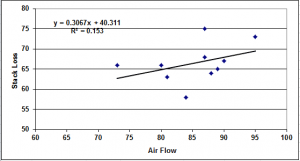# Six Sigma Regression Sum – Analysis of Variance (ANOVA) – Excel Download

## Six Sigma Regression Sum – ANOVA

If you don\’t have minitab, no problem. Microsoft Excel does provide powerful statistical analysis. Try this tool to get a simple regression sum ANOVA. While it\’s not as complete as what you would find in Minitab, you would be surprised by this complex Excel tool. This Excel template is multi-tabbed, and allows you to enter runs so that it will produce DF, SS, MS, F, and P results